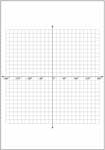1. Worksheets>
2. Math>
3. Geometry>
4. Graphing>
5. Graph Papers and Grids

# Printable Graph Papers and Grid Templates

This page contains a lot of printable graph papers and grids in all possible scales. Most of the Cartesian graph papers come up with three options, 'axes with labels', 'only axes' and 'only grids'. Also contains different coordinate systems like Cartesian, polar and trigonometric coordinates. Take a print out of some of these templates for free!

Standard Graph Paper

Download the graph paper pdfs and start graphing bars, functions, curves or anything you need. There are two sizes A3 and A4. Each size is available in four colors.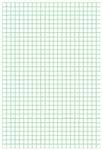A3 Size:

A4 Size:

Dot Graphing Paper

The distance between any two consecutive dots is the same. There are five choices in unit sizes.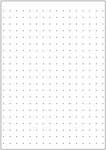5 by 5 Grid: Positive Quadrant (0 to 5)

The printable grid template consists of five rows and five columns. For the graph paper with no scale, you can use the scale increments by 1, 2, 5 or any multiples but only up to five numbers.Axes with labels:

Only Axes:

Only Grids:

10 by 10 Grid Paper

Cartesian graph paper with 10 rows and 10 columns. There are 10 'x numbering' and 10 'y numbering' possible in scale.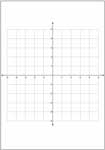Axes with labels:

Only Axes:

Only Grids:

Axes with labels:

Only Axes:

Only Grids:

15 by 15 Grid: Positive Quadrant (0 to 15)

Each graph paper contains 15 x 15 grid.Axes with labels:

Only Axes:

Only Grids:

20 by 20 Grid Paper

Each printable Cartesian graph sheet contains the grid with 40 squares.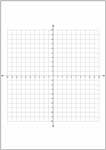With Axes and Labels:

With Axes but no Labels:

Only Grid:

With Axes and Labels:

With Axes but no Labels:

Only Grid:

25 by 25 Grid: Positive Quadrants (0 to 25)

The window size is 25 x 25. Only one grid is given in each sheet.Only Grids

Large Scale Grids

Some rarely used graph papers for functions with higher x and y values.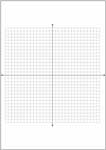30 by 30 - All Quadrants:

40 by 40 - All Quadrants:

50 by 50 - Positive Quadrant:

50 by 50 - All Quadrant:

100 by 100 - Positive Quadrant:

100 by 100 - All Quadrants:

Polar Graph Paper

Each polar graph paper contains 15 concentric circles that completely fit into the frame.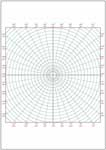Trigonometric Graph Paper: 0 to 2 pi

This bunch of graph paper pdfs helps you in plotting trigonometric functions.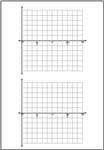0 to 2 pi (degrees):